## A recent pseudo-random number generator

R. Impagliazzo and M. Naor18proposed a pseudo-random number generator that involved only addition:

Choose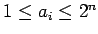randomly, for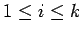, with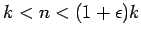. Choose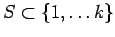randomly. Add thecorresponding tomodto obtain a sequence of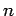bits. Use the first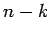bits as output from the generator. The remainingbits give you a new, for which you obtain a new sum.

This generator is more efficient than the one in section 8.1, since it uses simpler operations and produces more than one bit of pseudo-random output per iteration. The paper establishes connections between the existence of efficient tests (in the sense of section 8.3) and the average-case difficulty of subset-sum problems. We will not try to give a precise statement of the result, but will try to indicate some of the main ideas.

Suppose there were an efficient algorithmwhich looked at theand at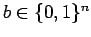and guessed whether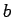came from a sum of thewith probability greater than. We will show that such an algorithm could be used to determine whethercame from a sum with probability close to 1.

In the case in whichwe show thatcan be used to guess, for each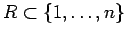, whetheris even or odd with probability greater than. Theorem 34 can then be used to identifywith probability close to 1.

To guess the parity of, we make a guess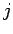for the size of this set and choose a random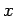. We havelook at a problem in whichis replaced by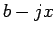andis replaced by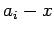for each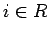. Ifis a correct guess,will see a sum of a subset. Otherwise,will be a random number, and we assumedcould distinguish between these two possibilities with probability greater than.

#### Footnotes

... M. Naor18
Efficient Cryptographic Schemes Provably as Secure as Subset Sum,'' Symposium on Foundations of Computer Science, 1989